# COMPUTING MARGINS OF ERROR IN OPINION POLLS

#### Jeffrey S. Rosenthal, August 2018

Note: Margins of error are discussed in a more accessible way in my 2005 book Struck by Lightning: The Curious World of Probabilities. See also my previous longer article.

Suppose you flip a coin ten thousand times. How many heads will you get? On each flip, the coin has equal probability of coming up heads or tails. So, on AVERAGE, you will get five thousand heads and five thousand tails. On the other hand, it doesn't seem likely that you will get EXACTLY five thousand heads -- rather, you will get "about" five thousand heads. But how much UNCERTAINTY is there around that mean value? That is, how far off from five thousand can we expect it to be? Might you get six thousand heads? Seven thousand? Or will you usually get between 4995 and 5005?

Meanwhile, we are constantly bombarded by the results of opinion polls. For example, a recent survey asked 5,000 Canadian adults if they consume cannabis recreationally, and found that 26% said yes. They asserted that their survey "has a margin of error of 1.4 per cent, 19 times out of 20". Similar claims are made all the time. What in the world do they mean? And, how do the polling firms reach these conclusions?

It turns out that these two questions, about coins and polls, are very closely related, and can both be understood fairly easy.

#### Coin Flipping Probabilities

Let's begin with coins. If you flip ten coins (say), then you might get exactly half heads, but you might not. In fact, the probabilities for the fraction of heads that you will get are as follows: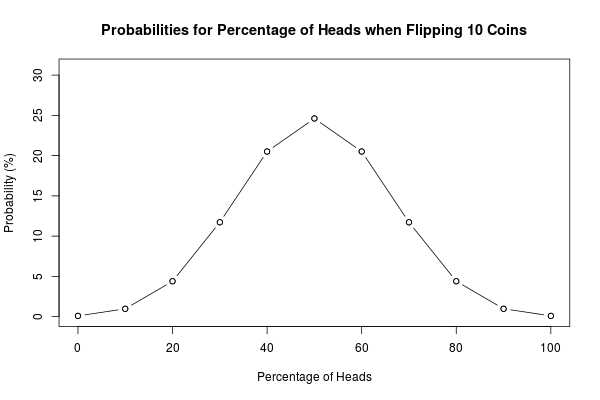That is, about 25% of the time you will get exactly 50% heads, but you have about a 20.5% chance of getting 40% heads, or an 11.7% chance of getting 70% heads, and so on.

If you flip 100 coins, the probabilities are as follows: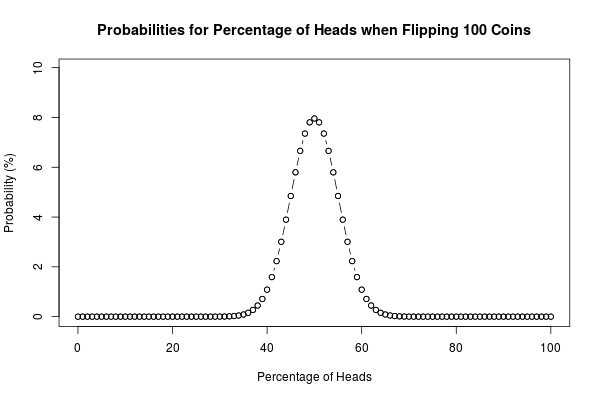This time, there is only an 8% chance of getting exactly 50% heads. On the other hand, the probabilities are much more concentrated near to 50% heads, and there is virtually no chance of getting less than 35% or more than 65% heads.

With 1000 coins, this concentration is even more pronounced: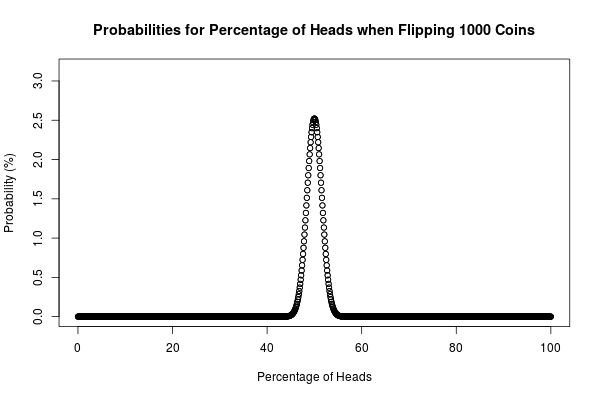There is now just a 2.5% chance of getting exactly 50% heads, but on the other hand there is virtually no chance of getting less than 45% or more than 55% heads.

Even more importantly, a pattern is starting to emerge. The shape of these probability graphs is becoming more and more like the bell curve or normal (or "Gaussian") distribution: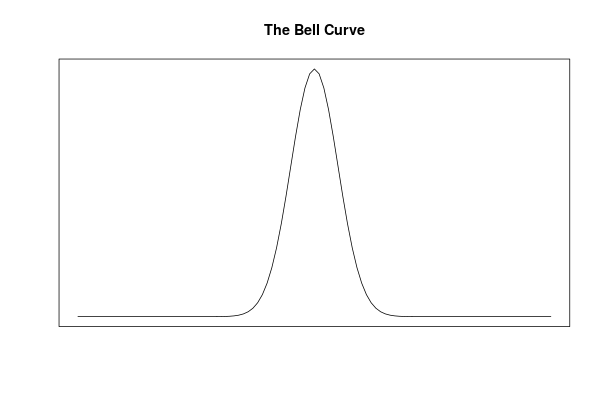Indeed, the central limit theorem tells us that when any random experiment (like flipping a coin) is repeated many times, the resulting probabilities will tend to follow this shape. And this is what allows us to compute margins of error!

#### Margins of Error for Coin Flipping

For the bell curve (or normal distribution), the probabilities are represented by the area under the graph. So, to figure out what will happen "19 times out of 20" (i.e., 95% of the time), we just have to figure out how large an interval is required to include 95% of the area under the graph. For a "standard-sized" (unit) normal distribution, the required interval goes from -196% to +196%: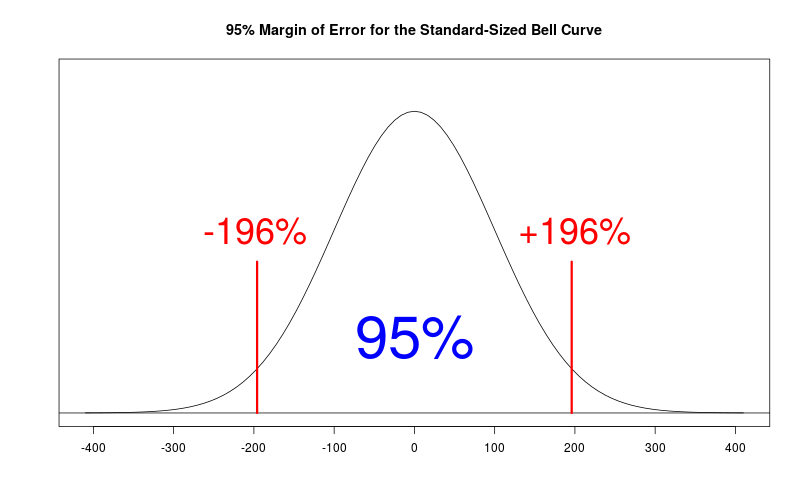For coin flipping, a bit of math shows that the fraction of heads has a "standard deviation" equal to one divided by twice the square root of the number of samples, i.e. to 1/2√n. So, to figure out the margin of error for the fraction of heads when flipping n coins, we simply have to multiply the above 196% by this standard deviation, to obtain the value 98%/√n, i.e. 98% divided the square root of the number of samples.

The conclusion is the following. If you flip n coins, then 95% of the time (i.e., 19 times out of 20), your fraction of heads will be within 98%/√n of the "true" answer of 50%. That is, it will be between 50% − 98%/√n, and 50% + 98%/√n.

#### Margins of Error for Polls

So how is all of this related to the claim that a survey of 5,000 people "has a margin of error of 1.4 per cent, 19 times out of 20"? Actually, it is essentially the same thing!

Conducting a survey involves phoning people at random to ask their opinion. Each call is sort of like a coin flip: conducting a random experiment, and recording the outcome. The main difference is that with a coin, we know that the probability of heads should be 50%, but with a survey we don't know the probability (indeed, that is what we are trying to figure out).

Nevertheless, we can use the same formula for the margin of error! We can still simply take 98%, and divide it by the square root of the number of samples.

For example, for a survey of n=5,000 people, the margin of error is 98%/√n = 98%/√5,000 = 1.385929% ≅ 1.4%. So, this is why we can say that the results of the survey will be accurate to within 1.4%, 19 times out of 20.

And that is how margins of error are computed! It's simple! The next time you see a poll, you can compute the margin of error yourself, and impress your friends!